Next: Hard Constraints Up: Mathematical Formulation Previous: Mathematical Formulation

## Full Dynamics

There are a wide variety of ways to model physical systems. The model needs to include parameters that describe the links that compose the system, as well as information about the hard constraints that connect these links to one another. A model that only includes this information is called a kinematic model, and can only describe the static states of a system. The state vector of a kinematic model consists of the model state,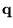.

A system in motion is more completely modeled when the dynamics of the system are modeled as well. A dynamic model describes the state evolution of the system over time. In a dynamic model the state vector includes velocity as well as position: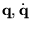. And state evolves according to Newton's First Law: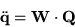Where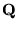is the vector of external forces applied to the system, and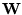is the inverse of the system mass matrix. The mass matrix describes the distribution of mass in the system.

1999-02-13